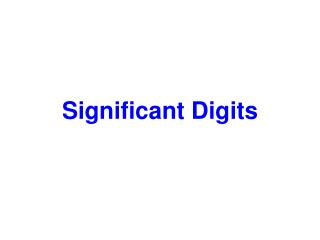DownloadDownload PresentationSignificant Digits

# Significant Digits

Download Presentation## Significant Digits

- - - - - - - - - - - - - - - - - - - - - - - - - - - E N D - - - - - - - - - - - - - - - - - - - - - - - - - - -
##### Presentation Transcript

1. Significant Digits

2. What are significant digits? The significant digits in a measurement consist of all the digits known with certainty plus one final digit, which is uncertain or is estimated.

3. For example: Study the diagram below. Using the ruler at the top of the diagram, what is the length of the darker rectangle found in between the two rulers? Answer: The length is between 4 and 5 cm. The “4” is certain, but the distance past 4 cm will have to be estimated. A possible estimate might be 4.3. Both of these digits are significant. The first digit is certain and th second digit is uncertain because it is an estimate.

4. Using the ruler at the bottom of the diagram, what is the length of the darker rectangle found in between the two rulers? Answer: The edge of the rectangle is between 4.2 cm and 4.3 cm. We are certain about the 4.2, but the next digit will have to be estimated. As possible estimation might be 4.27. All three digits would be significant. The first two digits are certain and the last digit is uncertain.

6. There are quite a few rules that determine how many significant digits a measurement has. You will need to memorize these rules.

7. RULE: If a number contains no zeros, all of the digits are significant. How many significant digits are in each of the following examples? a) 438 b) 26.42 c) 1.7 d) .653 Answers: a. b. c. d.

8. RULE: All zeros between two non zero digits are significant. How many significant digits are in each of the following examples? a) 506 b) 10,052 c) 900.431 Answers: a. b. c.

9. RULE: Zeros to the right of a non zero digita) If they are to the right of a nonzero number but not sandwiched between nonzero and decimal point, they are not significant. How many significant digits are in each of the following examples? a) 4830 b) 60 c) 4,000 Answers: a. b. c.

10. RULE: Zeros to the right of a non zero digitb) If these zeros are sandwiched between a nonzero number and a decimal point, they are significant. How many significant digits are in each of the following examples? a) 4830. b) 60. c) 4,000. Answers: a. b. c.

11. RULE: In decimals less than one, zeros to the right of a decimal point that are to the left of the first non-zero digit are never significant. They are simply place holders. How many significant digits are in each of the following examples? a) 0.06 b) 0.0047 c) 0.005 Answers: a. b. c.

12. RULE: All zeros to the right of a decimal point and to the right of a non-zero digit are significant. How many significant digits are in each of the following examples? a) .870 b) 8.0 c) 16.40 d) 35.000 e) 1.60 Answers: a. b. c. d. e.

13. Practice Problems How many significant digits are in each of the following examples? 1) 47.1 2) 9700. 3) 0.005965000 4) 560 5) 0.0509 6) 701.905 7) 50.00 8) 50.012 9) 0.000009 10) 0.0000104 Answers: 1. 2. 3. 4. 5. 6. 7. 8. 9. 10.

14. Determining Significant Digits When Rounding 1) 689.683 grams (4 significant digits) 2) 0.007219 (2 significant digits) 3) 4009 (1 significant digit) 4) 39.21 x 10-1 (1 significant digit) 5) 8792 (2 significant digits) 6) 309.00275 (5 significant digits) 7) .1046888 (3 significant digits) 1. 2. 3. 4. 5. 6. 7.

15. Rule for Addition and Subtraction When adding or subtracting, round the sum or difference so that it has the same number of decimal places as the measurement having the fewest decimal places. Example: Add 369.3389 + 17.24 First simply add the two numbers. Answer = 386.5789 17.24 had the fewest number of decimal places with 2 places past the decimal. The above answer will have to be rounded to two places past the decimal. Rounded Answer = 386.58

16. Find the sum or difference of the following and round them to the correct number of digits. a) 39.61 – 17.3 b) 1.97 + 2.700 c) 100.8 – 45 d) 296.0 + 3.9876 Answers a. b. c. d.

17. Rule for Multiplication and Division Express a product or a quotient to the same number of significant figures as the multiplied or divided measurement having the fewer significant figures. Example: Multiply 6.99 x .25 First simply multiply the two numbers. Answer = 1.7475 .25 had the fewest number of significant digits with 2. The above answer will have to be rounded to two significant digits. Rounded Answer = 1.7

18. Multiply or divide the following and give your answer in the correct number of significant digits. a) 4.7929  4.9 b) 5 x 3.999 c) 84  .09 d) .815 x 215.7 Answers a. b. c. d.